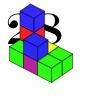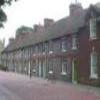Search by Topic

Resources tagged with Properties of numbers similar to Prime Magic:

Filter by: Content type:
Age range:
Challenge level:

There are 63 results

Broad Topics > Numbers and the Number System > Properties of numbersPrime Magic

Age 7 to 16 Challenge Level:

Place the numbers 1, 2, 3,..., 9 one on each square of a 3 by 3 grid so that all the rows and columns add up to a prime number. How many different solutions can you find?Multiply the Addition Square

Age 11 to 14 Challenge Level:

If you take a three by three square on a 1-10 addition square and multiply the diagonally opposite numbers together, what is the difference between these products. Why?Summing Consecutive Numbers

Age 11 to 14 Challenge Level:

15 = 7 + 8 and 10 = 1 + 2 + 3 + 4. Can you say which numbers can be expressed as the sum of two or more consecutive integers?Cogs

Age 11 to 14 Challenge Level:

A and B are two interlocking cogwheels having p teeth and q teeth respectively. One tooth on B is painted red. Find the values of p and q for which the red tooth on B contacts every gap on the. . . .Take One Example

Age 5 to 11

This article introduces the idea of generic proof for younger children and illustrates how one example can offer a proof of a general result through unpacking its underlying structure.Age 7 to 14 Challenge Level:

I added together some of my neighbours' house numbers. Can you explain the patterns I noticed?Special Numbers

Age 11 to 14 Challenge Level:

My two digit number is special because adding the sum of its digits to the product of its digits gives me my original number. What could my number be?Got it Article

Age 7 to 14

This article gives you a few ideas for understanding the Got It! game and how you might find a winning strategy.Magic Letters

Age 11 to 14 Challenge Level:

Charlie has made a Magic V. Can you use his example to make some more? And how about Magic Ls, Ns and Ws?Light the Lights Again

Age 7 to 11 Challenge Level:

Each light in this interactivity turns on according to a rule. What happens when you enter different numbers? Can you find the smallest number that lights up all four lights?Always, Sometimes or Never? Number

Age 7 to 11 Challenge Level:

Are these statements always true, sometimes true or never true?Cinema Problem

Age 11 to 14 Challenge Level:

A cinema has 100 seats. Show how it is possible to sell exactly 100 tickets and take exactly £100 if the prices are £10 for adults, 50p for pensioners and 10p for children.28 and It's Upward and Onward

Age 7 to 11 Challenge Level:

Can you find ways of joining cubes together so that 28 faces are visible?Babylon Numbers

Age 11 to 18 Challenge Level:

Can you make a hypothesis to explain these ancient numbers?Sort Them Out (2)

Age 7 to 11 Challenge Level:

Can you each work out the number on your card? What do you notice? How could you sort the cards?Oh! Hidden Inside?

Age 11 to 14 Challenge Level:

Find the number which has 8 divisors, such that the product of the divisors is 331776.Table Patterns Go Wild!

Age 7 to 11 Challenge Level:

Nearly all of us have made table patterns on hundred squares, that is 10 by 10 grids. This problem looks at the patterns on differently sized square grids.Which Numbers? (1)

Age 7 to 11 Challenge Level:

I am thinking of three sets of numbers less than 101. They are the red set, the green set and the blue set. Can you find all the numbers in the sets from these clues?Thirty Six Exactly

Age 11 to 14 Challenge Level:

The number 12 = 2^2 � 3 has 6 factors. What is the smallest natural number with exactly 36 factors?Which Numbers? (2)

Age 7 to 11 Challenge Level:

I am thinking of three sets of numbers less than 101. Can you find all the numbers in each set from these clues?Even So

Age 11 to 14 Challenge Level:

Find some triples of whole numbers a, b and c such that a^2 + b^2 + c^2 is a multiple of 4. Is it necessarily the case that a, b and c must all be even? If so, can you explain why?Small Change

Age 11 to 14 Challenge Level:

In how many ways can a pound (value 100 pence) be changed into some combination of 1, 2, 5, 10, 20 and 50 pence coins?Three Neighbours

Age 7 to 11 Challenge Level:

Look at three 'next door neighbours' amongst the counting numbers. Add them together. What do you notice?Can You Find a Perfect Number?

Age 7 to 14

Can you find any perfect numbers? Read this article to find out more...Helen's Conjecture

Age 11 to 14 Challenge Level:

Helen made the conjecture that "every multiple of six has more factors than the two numbers either side of it". Is this conjecture true?Arrange the Digits

Age 11 to 14 Challenge Level:

Can you arrange the digits 1,2,3,4,5,6,7,8,9 into three 3-digit numbers such that their total is close to 1500?X Marks the Spot

Age 11 to 14 Challenge Level:

When the number x 1 x x x is multiplied by 417 this gives the answer 9 x x x 0 5 7. Find the missing digits, each of which is represented by an "x" .Counting Factors

Age 11 to 14 Challenge Level:

Is there an efficient way to work out how many factors a large number has?Satisfying Statements

Age 11 to 14 Challenge Level:

Can you find any two-digit numbers that satisfy all of these statements?Chameleons

Age 11 to 14 Challenge Level:

Whenever two chameleons of different colours meet they change colour to the third colour. Describe the shortest sequence of meetings in which all the chameleons change to green if you start with 12. . . .Mini-max

Age 11 to 14 Challenge Level:

Consider all two digit numbers (10, 11, . . . ,99). In writing down all these numbers, which digits occur least often, and which occur most often ? What about three digit numbers, four digit numbers. . . .Unit Fractions

Age 11 to 14 Challenge Level:

Consider the equation 1/a + 1/b + 1/c = 1 where a, b and c are natural numbers and 0 < a < b < c. Prove that there is only one set of values which satisfy this equation.One to Eight

Age 11 to 14 Challenge Level:

Complete the following expressions so that each one gives a four digit number as the product of two two digit numbers and uses the digits 1 to 8 once and only once.Age 11 to 14 Challenge Level:

Visitors to Earth from the distant planet of Zub-Zorna were amazed when they found out that when the digits in this multiplication were reversed, the answer was the same! Find a way to explain. . . .Numbers Numbers Everywhere!

Age 5 to 11

Bernard Bagnall recommends some primary school problems which use numbers from the environment around us, from clocks to house numbers.What Are Numbers?

Age 7 to 18

Ranging from kindergarten mathematics to the fringe of research this informal article paints the big picture of number in a non technical way suitable for primary teachers and older students.Four Coloured Lights

Age 11 to 14 Challenge Level:

Imagine a machine with four coloured lights which respond to different rules. Can you find the smallest possible number which will make all four colours light up?Whole Numbers Only

Age 11 to 14 Challenge Level:

Can you work out how many of each kind of pencil this student bought?The Patent Solution

Age 11 to 14 Challenge Level:

A combination mechanism for a safe comprises thirty-two tumblers numbered from one to thirty-two in such a way that the numbers in each wheel total 132... Could you open the safe?Six Times Five

Age 11 to 14 Challenge Level:

How many six digit numbers are there which DO NOT contain a 5?Alphabet Soup

Age 11 to 14 Challenge Level:

This challenge is to make up YOUR OWN alphanumeric. Each letter represents a digit and where the same letter appears more than once it must represent the same digit each time.Elevenses

Age 11 to 14 Challenge Level:

How many pairs of numbers can you find that add up to a multiple of 11? Do you notice anything interesting about your results?The Codabar Check

Age 11 to 14

This article explains how credit card numbers are defined and the check digit serves to verify their accuracy.Times Right

Age 11 to 16 Challenge Level:

Using the digits 1, 2, 3, 4, 5, 6, 7 and 8, mulitply a two two digit numbers are multiplied to give a four digit number, so that the expression is correct. How many different solutions can you find?Palindromes

Age 5 to 14Happy Octopus

Age 11 to 14 Challenge Level:

This investigation is about happy numbers in the World of the Octopus where all numbers are written in base 8 ... Find all the fixed points and cycles for the happy number sequences in base 8.Magic Crosses

Age 7 to 14 Challenge Level:

Can you find examples of magic crosses? Can you find all the possibilities?Power Crazy

Age 11 to 14 Challenge Level:

What can you say about the values of n that make $7^n + 3^n$ a multiple of 10? Are there other pairs of integers between 1 and 10 which have similar properties?Like Powers

Age 11 to 14 Challenge Level:

Investigate $1^n + 19^n + 20^n + 51^n + 57^n + 80^n + 82^n$ and $2^n + 12^n + 31^n + 40^n + 69^n + 71^n + 85^n$ for different values of n.Not a Polite Question

Age 11 to 14 Challenge Level:

When asked how old she was, the teacher replied: My age in years is not prime but odd and when reversed and added to my age you have a perfect square...